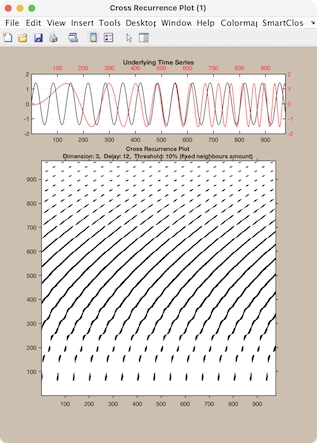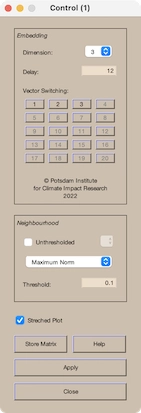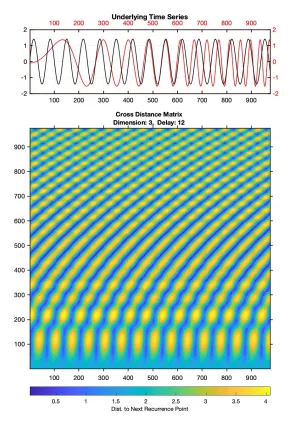# CRP Toolbox

## crp

Creates a cross recurrence plot/ recurrence plot or a distance matrix/ order matrix.

### Syntax

``````crp(x)
crp(x,y)
crp(x,m,t,e)
r=crp(x,[],m,t,e)
r=crp(x,m,t,e,'param1','param2',...)
r=crp(x,y,m,'param1')
``````

### Description

Creates a cross recurrence plot/ recurrence plot. Results can be stored into the workspace.

Allows to change the parameters interactively by using a GUI.

The source-data x and test-data y can be one- or a two-coloumn vectors (then, in the first column have to be the time); if the test-data y is not specified, a simple (auto) recurrence plot is created.### Parameters

Dimension m, delay t and the size of neighbourhood e are the first three numbers after the data series; further parameters can be used to switch between various methods of finding the neighbours of the phasespace trajectory, to suppress the normalization of the data and to suppress the GUI (useful in order to use this programme by other programmes).

 Methods of finding the neighbours/ of plot. 'maxnorm' - Maximum norm. 'euclidean' - Euclidean norm. 'minnorm' - Minimum norm. 'nrmnorm' - Euclidean norm between normalized vectors(all vectors have the length one). 'rr' - Maximum norm, fixed recurrence rate. 'fan' - Fixed amount of nearest neighbours. 'inter' - Interdependent neighbours. 'omatrix' - Order matrix. 'opattern' - Order patterns recurrence plot. 'distance' - Distance coded matrix (global CRP, Euclidean norm). Normalization of the data series. 'normalize' - Normalization of the data. 'nonormalize' - No normalization of the data. Suppressing the GUI. 'gui' - Creates the GUI and the output plot. 'nogui' - Suppresses the GUI and the output plot. 'silent' - Suppresses all output.

Parameters are optional and not required.

### Limitations

For higher speed in output the whole matrix of the recurrence plot is in the work space - this limits the application of long data series. However, with a little Matlab script, long data series can be handled too (cf. Examples).

### Examples

``````a=sin((1:1000)*2*pi/67);
crp(a,'nonorm','euclidean')

X=crp(a,2,50,.1,'nogui');
spy(double(X))

b=sin(.01*([1:1000]*2*pi/67).^2);
crp(a,b,3,12,'distance')
``````For computing RPs/ CRPs of long data series, use a similar script as in the following. The data length is finally limited by the used platform performance. The examples also illustrate the capability of using the programms in a script. The first example uses sparse matrices:

``````m=3; t=20; e=.5; w=300;
x1=sin((1:5000)/40)'; x2=sin((1:7000)/80)';

clear Y, Y=spalloc(length(x2)-(m-1)*t,length(x1)-(m-1)*t,1);
k=0; h1=waitbar(0,'Compute sub CRPs - Please be patient.');
Nx=length(x1)-(m-1)*t; Ny=length(x2)-(m-1)*t;
ax=ceil(Nx/w); ay=ceil(Ny/w);
Nx2=floor(Nx/ax); Ny2=floor(Ny/ay);
for i=1:Nx2:Nx-Nx-2;
for j=1:Ny2:Ny-Ny-2, k=k+1; waitbar(k/(Nx*Ny/(Nx2*Ny2)))
X2=crp(x1(i:i+Nx2+(m-1)*t), x2(j:j+Ny2+(m-1)*t),m,t,e,...
'nonorm','max','silent');
X=sparse(double(X2));
Y(j:j+Ny2-1, i:i+Nx2-1)=X(1:Ny2,1:Nx2);
end
end
close(h1)

spy(Y)
``````

The second example writes single RPs/ CRPs to the hard disk:

``````m=3; t=20; e=.5; w=300;
x1=sin((1:5000)/40)'; x2=sin((1:7000)/80)';
Nx=length(x1); Ny=length(x2);

% compute single CRPs and write them to the hard disk
b1=zeros((m-1)*t+ceil(length(x1)/w)*w,1);
b1(1:length(x1))=x1;
b2=zeros((m-1)*t+ceil(length(x2)/w)*w,1);
b2(1:length(x2))=x2;
h=waitbar(0,'Compute sub CRPs - Please be patient.')
for i=1:w:length(b1)-w-1, waitbar(i/((length(b1)-w-1)))
for j=1:w:length(b2)-w-1,j
X=crp(b1(i:i+w+(m-1)*t-1),b2(j:j+w+(m-1)*t-1),m,t,e,...
'max','silent','nonorm');
i2=num2str((i+w-1)/w);j2=num2str((j+w-1)/w);
filename=['CRP_',i2,'_',j2,'.tif'];
imwrite(X,filename,'tif')
end
end, close(h)

% read single CRPs and unify them
xmax=(i+w-1)/w; ymax=(j+w-1)/w;
for i=1:xmax,waitbar(i/xmax)
for j=1:ymax,
i2=num2str(i);j2=num2str(j);
filename=['CRP_',i2,'_',j2,'.tif'];